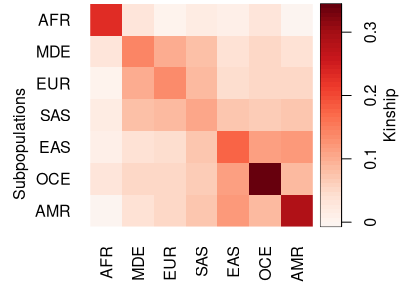# Estimate Kinship and FST under Arbitrary Population Structure with popkin

#### 2021-07-27

$\newcommand{\Fst}{F_{\text{ST}}} \newcommand{\ft}[j]{f_{#1}^T} \newcommand{\kt}[k]{\varphi_{j#1}^T}$

# Introduction

The popkin (“population kinship”) package estimates the kinship matrix of individuals and $$\Fst$$ from their biallelic genotypes. Our estimation framework is the first to be practically unbiased under arbitrary population structures (Ochoa and Storey 2021, 2016). We have also applied our approach to real human datasets (Ochoa and Storey 2019). Here we briefly summarize the notation and intuition behind the key parameters.

## Kinship and inbreeding coefficients

Kinship and inbreeding coefficients are probabilities of “identity by descent” (IBD) carefully defined elsewhere (Ochoa and Storey 2021, 2016). The reference ancestral population $$T$$ sets the level of relatedness treated as zero (as demonstrated in the sample usage section below). $$\ft$$ is the inbreeding coefficient of individual $$j$$ when $$T$$ is the ancestral population, and $$\kt$$ is the kinship coefficient of the pair individuals $$j,k$$ when $$T$$ is the ancestral population. In a structured population we expect most $$\ft,\kt >0$$. If $$j,k$$ are the parents of $$l$$ then $$\ft[l] = \kt$$, so within a panmictic subpopulation we expect $$\ft \approx \kt$$ for $$j \ne k$$. The “self-kinship” $$j=k$$ case equals $$\kt[j] = \frac{1}{2}\left( 1+\ft \right)$$ rather than $$\ft$$.

Let $$\Phi^T = (\kt)$$ be the $$n \times n$$ matrix that contains all kinship coefficients of all individuals in a dataset. The ancestral population $$T$$ is the most recent common ancestor (MRCA) population if and only if $$\min \kt = 0$$, assuming such unrelated pairs of individuals exist in the dataset. Thus, the only role $$T$$ plays in our estimates is determining the level of relatedness that is treated as zero.

Note that the diagonal of our estimated $$\Phi^T$$ contains $$\kt[j]$$ values rather than $$\ft$$, which is required for statistical modeling applications; however, $$\kt[j]$$ tends to take on much greater values than $$\kt$$ for $$j \ne k$$, while $$\ft \approx \kt$$ for $$j \ne k$$ within panmictic subpopulations (see above), so for visualization we strongly recommend replacing the diagonal of $$\Phi^T$$ with $$\ft$$ values.

## The generalized $$\Fst$$

$$\Fst$$ is also an IBD probability that equals the mean inbreeding coefficients in a population partitioned into homogeneous subpopulations. We recently generalized the $$\Fst$$ definition to arbitrary population structures—dropping the need for subpopulations—and generalized the partition of “total” inbreeding into “local” inbreeding (due to having unusually closely related parents) and “structural” inbreeding (due to the population structure) (Ochoa and Storey 2016). The current popkin estimates the total kinship matrix $$\Phi^T$$ only; in the future, popkin will also extract the structural kinship matrix. However, when all individuals are “locally outbred”—the most common case in population data—$$\Fst$$ is simply the weighted mean inbreeding coefficient: $\Fst = \sum_{j=1}^n w_j \ft,$ where $$0 < w_j < 1, \sum_{j=1}^n w_j = 1$$ are weights for individuals intended to help users balance skewed samples (i.e. if there are subpopulations with much greater sample sizes than others). The current popkin version assumes all individuals are locally outbred in estimating $$\Fst$$.

## The individual-level pairwise $$\Fst$$

Another quantity of interest is the individual-level pairwise $$\Fst$$, which generalize the $$\Fst$$ between two populations to pairs of individuals. Here each comparison between two individuals has a different ancestral population, namely the MRCA population of the two individuals. When individuals are again locally outbred and also locally unrelated, the pairwise $$\Fst$$ is given in terms of the inbreeding and kinship coefficients (Ochoa and Storey 2016): $F_{jk} = \frac{\frac{\ft+\ft[k]}{2}-\kt}{1-\kt}.$ The popkin package also provides an estimator of the pairwise $$\Fst$$ matrix (containing $$F_{jk}$$ estimates between every pair of individuals).

# Sample usage

## Input genotype data

The popkin function accepts biallelic genotype matrices in three forms:

1. A genotype matrix X with values in c(0,1,2,NA) only. It is preferable, though not necessary, for X to be an integer matrix (with values in c(0L,1L,2L,NA) only, see ?storage.mode). This standard encoding for biallelic SNPs counts reference alleles: 2 is homozygous for the reference allele, 0 is homozygous for the alternative allele, 1 is heterozygous, and NA is missing data. Which allele is the reference does not matter: popkin estimates the same kinship and $$\Fst$$ for X and 2-X. By default popkin expects loci along rows and individuals along columns (an $$m \times n$$ matrix); a transposed X is handled best by also setting loci_on_cols = TRUE.

2. BED-formatted data loaded with the BEDMatrix package, which popkin uses to keep memory usage low. For example, load myData.bed, myData.bim, myData.fam using:

library(BEDMatrix)
X <- BEDMatrix('myData') # note: excluding extension is ok

This BEDMatrix object is not a regular matrix but popkin handles it correctly. Other genotype formats can be converted into BED using plink2 or other software.

1. A function X(m) that when called loads the next $$m$$ SNPs of the data, returning an $$m \times n$$ matrix in the format of Case 1 above. This option allows direct and memory-efficient processing of large non-BED data, but should be the last resort since users must write their own functions X(m) for their custom formats. Try first converting your data to BED and loading with BEDMatrix.

## Load and clean sample data

For illustration, let’s load the real human data worldwide sample (“HGDP subset”) contained in the lfa package:

library(popkin)
# for hgdp_subset sample data only
library(lfa)
# rename for simplicity
X <- hgdp_subset
dim(X)
#>  5000  159

This data has $$m=5000$$ loci and $$n=159$$ individuals, and is oriented as popkin expects by default. These samples have labels grouping them by continental subpopulation in colnames(X). To make visualizations easier later on, let’s shorten these labels and reorder to have nice blocks:

# shorten subpopulation labels
colnames(X)[colnames(X) == 'AFRICA'] <- 'AFR'
colnames(X)[colnames(X) == 'MIDDLE_EAST'] <- 'MDE'
colnames(X)[colnames(X) == 'EUROPE'] <- 'EUR'
colnames(X)[colnames(X) == 'CENTRAL_SOUTH_ASIA'] <- 'SAS'
colnames(X)[colnames(X) == 'EAST_ASIA'] <- 'EAS'
colnames(X)[colnames(X) == 'OCEANIA'] <- 'OCE'
colnames(X)[colnames(X) == 'AMERICA'] <- 'AMR'
# order roughly by distance from Africa
# (without crossing the Atlantic Ocean)
pop_order <- c('AFR', 'MDE', 'EUR', 'SAS', 'EAS', 'OCE', 'AMR')
# applies reordering
X <- X[, order(match(colnames(X), pop_order))]
# extract subpopulations vector
subpops <- colnames(X)

Here’s a quick view of the top left corner of the matrix X with values in 0, 1, 2, and NA (this example has no missing values, but popkin handles them too). This matrix does not preserve the identity of the reference or alternative alleles, but this distinction does not matter for estimating kinship and $$\Fst$$.

X[1:10,1:15]
#>            AFR AFR AFR AFR AFR AFR AFR AFR AFR AFR AFR AFR AFR AFR AFR
#> rs4050954    2   2   1   2   1   1   1   2   2   0   0   2   0   2   0
#> rs302665     0   0   0   0   0   0   2   0   0   1   0   0   0   0   0
#> rs2899446    2   1   2   2   1   2   1   2   2   2   2   1   2   2   2
#> rs10069940   2   1   2   2   0   2   0   0   1   0   0   0   0   0   1
#> rs6114921    1   0   0   1   2   1   2   2   1   0   1   1   0   0   1
#> rs201718     2   2   2   2   2   2   2   1   2   1   2   1   2   2   2
#> rs1335715    0   1   0   1   1   0   1   1   2   0   1   1   0   0   0
#> rs17006588   2   0   0   1   0   0   1   0   0   1   1   2   0   0   0
#> rs3885909    0   0   0   0   0   0   0   1   2   0   0   0   1   0   0
#> rs986457     0   1   0   1   1   0   2   1   1   1   1   1   1   1   0

Now we’re ready to analyze this data with popkin!

## Estimate and visualize kinship using genotypes and subpopulations

Estimating a kinship matrix requires the genotype matrix X and subpopulation levels used only to estimate the minimum level of kinship. Using the lfa sample data we cleaned in the last subsection, obtaining the estimate is simple:

kinship <- popkin(X, subpops)

Now let’s visualize the raw kinship matrix estimate:

plot_popkin(
kinship,
labs = subpops,
# shared bottom and left margin value, to make space for labels
mar = 1
)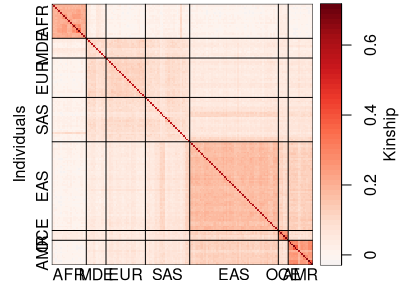Ignoring the overlapping labels for a moment, this plot shows that self-kinship (the diagonal) is much greater than kinship between different individuals (min $$\kt[j] \ge 0.5$$). It makes more sense to plot inbreeding ($$\ft$$) values on the diagonal (they are on the same scale as $$\kt$$ for $$j \ne k$$), which is achieved using inbr_diag:

plot_popkin(
inbr_diag( kinship ),
labs = subpops,
mar = 1
)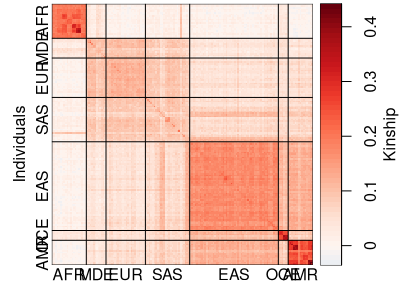Now let’s tweak the plot. We improve the labeling by setting labs_even = TRUE, which arranges the subpopulation labels with equal spacing and adds lines that map to their blocks. To see these new lines, we must move these labels further from the heatmap by setting labs_line = 1. We shrink the labels with labs_cex = 0.7.

plot_popkin(
inbr_diag(kinship),
labs = subpops,
labs_even = TRUE,
labs_line = 1,
labs_cex = 0.7,
mar = 2
)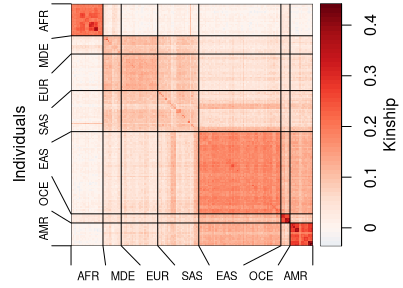This figure clearly shows the population structure of these worldwide samples, with block patterns that are coherent with serial founder effects in the dispersal of humans out of Africa. Since only $$m = 5000$$ SNPs are included in this sample, the estimates are noisier than in more complete data (datasets routinely have over 300K SNPs).

This figure also illustrates how subpopulations are used to estimate kinship by popkin: they only set the zero kinship as the mean kinship between the two most distant populations, which in this case are AFR and AMR.

## Estimate $$\Fst$$ and individual inbreeding from a kinship matrix

Since $$\Fst$$ is the weighted mean of the inbreeding coefficients, and since some subpopulations are overrepresented in this data (EAS is much larger than the rest), it makes sense to use weights that balance these subpopulations:

# get weights
w <- weights_subpops(subpops)
# compute FST!
# Note: don't use the output to inbr_diag(kinship) or FST will be wrong!
fst(kinship, w)
#>  0.2126638

If you compare these estimates to those we obtained for Human Origins (Ochoa and Storey 2016), you’ll notice things look a bit different: here $$\Fst$$ is smaller and the kinship within AFR is relatively much higher than within EUR or EAS. Besides containing many fewer SNPs, the SNPs in this HGDP sample were likely biased for common variants in Europeans, which might explain the difference.

We can also extract the vector of inbreeding coefficients from the kinship matrix using inbr:

# vector of inbreeding coefficients
inbrs <- inbr(kinship)
# quick plot
par(mar = c(4, 4, 0, 0.2) + 0.2)
# see their distribution
plot(density(inbrs), xlab = 'inbreeding coefficient', main = '')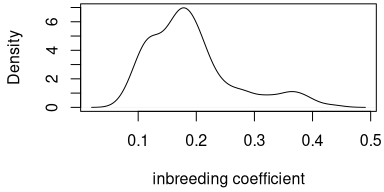## Estimate individual-level pairwise $$\Fst$$ matrix from a kinship matrix

We calculate individual-level pairwise $$\Fst$$ estimates from the previous kinship estimates using pwfst. Note that the pairwise $$\Fst$$ is a distance between pairs of individuals: approximately zero for individuals in the same population, and increasing for more distant pairs of individuals.

# compute pairwise FST matrix from kinship matrix
pairwise_fst <- pwfst(kinship)
# fancy legend label
leg_title <- expression(paste('Pairwise ', F[ST]))
# NOTE no need for inbr_diag() here!
plot_popkin(
pairwise_fst,
labs = subpops,
labs_even = TRUE,
labs_line = 1,
labs_cex = 0.7,
leg_title = leg_title,
mar = c(2, 0.2)
)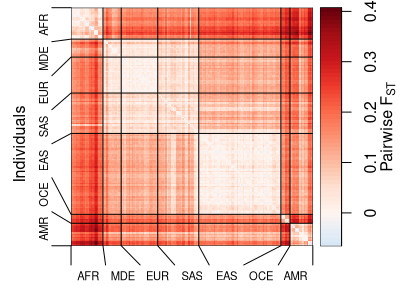## Rescale kinship matrix in a subset of the data

Suppose now you’re interested in one subpopulation, say AFR:

indexes_AFR <- subpops == 'AFR'
# AFR subset of the kinship matrix
kinship_AFR <- kinship[indexes_AFR, indexes_AFR]

# kinship matrix plot
plot_popkin(
inbr_diag( kinship_AFR ),
mar = 0
)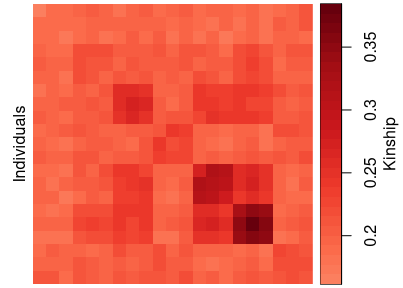# estimate FST before rescaling (this value will be wrong, too high!)
fst( kinship_AFR )
#>  0.2474861

Removing populations changes the MRCA population $$T$$, drastically in this case (the reason the minimum kinship is so large and the within-AFR $$\Fst$$ above is wrong). To ensure the minimum kinship is zero, instead of re-estimate the kinship matrix from the subset genotypes, it suffices to rescale the given kinship matrix with rescale_popkin!

# rescale kinship_AFR
# since subpops is missing, minimum kinship value is set to zero
# (no averaging between subpopulations)
kinship_AFR <- rescale_popkin( kinship_AFR )

# kinship matrix plot
plot_popkin(
inbr_diag( kinship_AFR ),
mar = c(0, 0.3)
)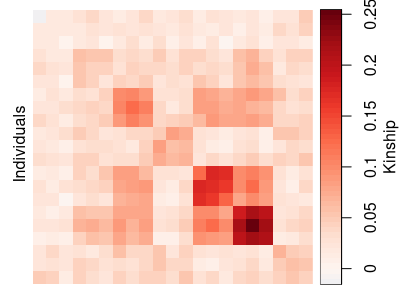# FST is now correct, relative to the MRCA of AFR individuals
fst( kinship_AFR )
#>  0.08879701

There is clear substructure within Sub-Saharan Africa, but this sample data does not have more detailed labels that could help us interpret further.

## Plot multiple kinship matrices together

As a final example, we plot the global kinship and the rescaled AFR subset kinship_AFR side-by-side, illustrating how more than one kinship matrix can be plotted with a shared legend.

# dummy labels to have lines in second panel
subpops_AFR <- subpops[ indexes_AFR ]
plot_popkin(
# inbr_diag also works on a list of matrices
inbr_diag( list( kinship, kinship_AFR ) ),
# title of each panel
titles = c('All', 'AFR only, rescaled'),
# pass per-panel labels using a list
labs = list( subpops, subpops_AFR ),
# scalar options are shared across panels
labs_even = TRUE,
labs_line = 1,
labs_cex = 0.5,
# second value is top margin (to make space for titles)
mar = c(2, 2)
)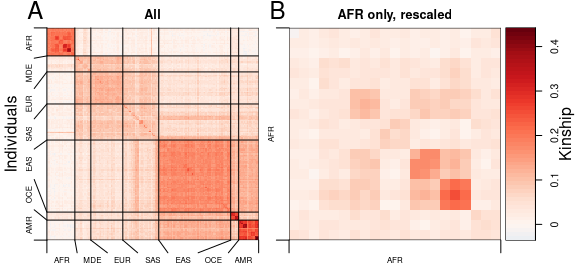## Plot kinship matrices with multiple levels of labels

The plot_popkin function has advanced options for plotting more than one level of labels. For this example, we will highlight the three “blocks” that represent the first two splits in the human migration out of Africa:

# create second level of labels
# first copy first-level labels
blocks <- subpops
# first block is AFR
blocks[blocks == 'AFR'] <- 'B1'
# second block is West Eurasians, broadly defined
blocks[blocks == 'MDE'] <- 'B2'
blocks[blocks == 'EUR'] <- 'B2'
blocks[blocks == 'SAS'] <- 'B2'
# third block is East Eurasians, broadly defined
blocks[blocks == 'EAS'] <- 'B3'
blocks[blocks == 'OCE'] <- 'B3'
blocks[blocks == 'AMR'] <- 'B3'

# plotting with different options per level is more complicated...
plot_popkin(
inbr_diag( kinship ),
labs = cbind( subpops, blocks ), # ... labs is now a matrix with levels on columns
labs_even = c(TRUE, FALSE),      # ... even spacing for first level only
labs_line = c(1, 2),             # ... put second level further out
labs_cex = c(0.7, 1),            # ... don't shrink second level
labs_sep = c(FALSE, TRUE),       # ... draw lines inside heatmap for second level only
ylab_adj = 0.65,                 # push up outer margin ylab "Individuals"
mar = 3                          # increase margins again
)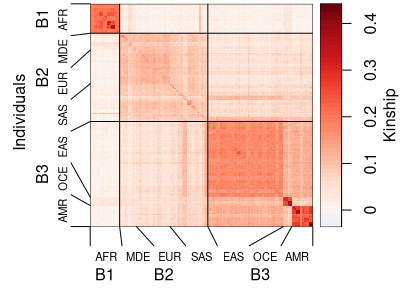Now we add a second panel to what we have above, showing how options must be passed when labels differ per panel and there are multiple levels:

plot_popkin(
inbr_diag(list(kinship, kinship_AFR)),
titles = c('All', 'AFR only, rescaled'),
# list of matrices
labs = list( cbind(subpops, blocks), subpops_AFR ),
# non-list: values are reused for both panels
labs_even = c(TRUE, FALSE),
labs_line = c(1, 2),
# make label bigger in second panel (custom per-panel values)
# list of vectors
labs_cex = list(c(0.5, 0.7), 1),
# add lines for first level of second panel (custom per-panel values)
# list of vectors
labs_sep = list(c(FALSE, TRUE), TRUE),
mar = c(3, 2)
)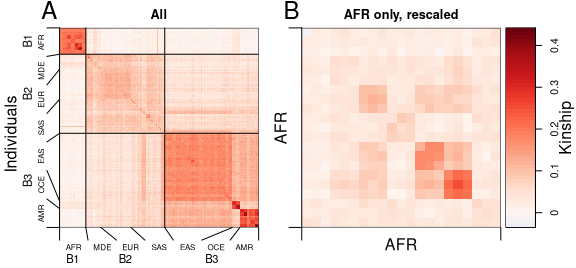Each panel can also have its own scale:

plot_popkin(
inbr_diag(list(kinship, kinship_AFR)),
titles = c('All', 'AFR only, rescaled'),
labs = list( cbind(subpops, blocks), subpops_AFR ),
labs_even = c(TRUE, FALSE),
labs_line = c(1, 2),
labs_cex = list(c(0.5, 0.7), 1),
labs_sep = list(c(FALSE, TRUE), TRUE),
mar = c(3, 2),
# this option adds a legend per panel
leg_per_panel = TRUE
)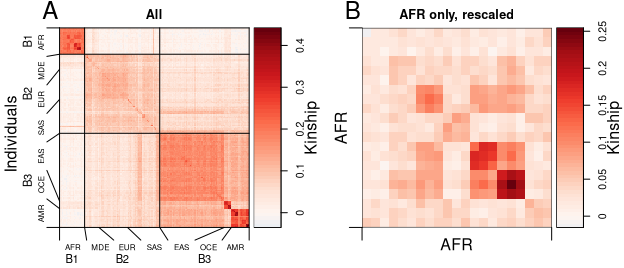## Coancestry estimation from allele frequencies

Analogously to the way popkin estimates kinship from genotype matrices, popkin_af estimates coancestry from allele frequency matrices. For this demonstration, we estimate allele frequencies from the HGDP data. However, this approach is most useful for data that does not have corresponding genotypes, such as the P matrices from admixture inference approaches.

This code creates the desired allele frequency matrix P, where each column belongs to a continental subpopulation

# number of loci (rows)
m_loci <- nrow( X )
# number of subpopulations (columns)
k_subpops <- length( pop_order )
# initialize matrix
P <- matrix( NA, nrow = m_loci, ncol = k_subpops )
# copy names from data
colnames( P ) <- pop_order
rownames( P ) <- rownames( X )
# navigate subpopulations
for ( u in 1 : k_subpops ) {
# subpopulation label name
pop <- pop_order[ u ]
# columns of interest
js <- subpops == pop
# now average genotypes into allele frequency estimates and store
P[ , u ] <- rowMeans( X[ , js ], na.rm = TRUE ) / 2
}

Now we use popkin_af to estimate the coancestry matrix:

coancestry <- popkin_af( P )

Lastly, we visualize this estimate, which resembles the kinship matrix estimated from individuals except it has much lower resolution:

# coancestry matrix plot
# NOTE: inbr_diag() is not needed for coancestry!
plot_popkin(
coancestry,
mar = 3,
names = TRUE,
ylab = 'Subpopulations'
)